# Time Constant Circuits

## DC Electric Circuits

• #### Question 1

Generally speaking, how many “time constants” worth of time does it take for the voltage and current to ßettle” into their final values in an RC or LR circuit, from the time the switch is closed?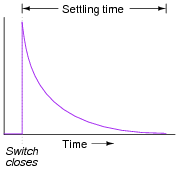• #### Question 2

Suppose a fellow electronics technician approaches you with a design problem. He needs a simple circuit that outputs brief pulses of voltage every time a switch is actuated, so that a computer receives a single pulse signal every time the switch is actuated, rather than a continuous ön” signal for as long as the switch is actuated:The technician suggests you build a passive differentiator circuit for his application. You have never heard of this circuit before, but you probably know where you can research to find out what it is! He tells you it is perfectly okay if the circuit generates negative voltage pulses when the switch is de-actuated: all he cares about is a single positive voltage pulse to the computer each time the switch actuates. Also, the pulse needs to be very short: no longer than 2 milliseconds in duration.

Given this information, draw a schematic diagram for a practical passive differentiator circuit within the dotted lines, complete with component values.

• #### Question 3

When a circuit designer needs a circuit to provide a time delay, he or she almost always chooses an RC circuit instead of an LR circuit. Explain why this is.

• #### Question 4

 Don’t just sit there! Build something!!

Learning to mathematically analyze circuits requires much study and practice. Typically, students practice by working through lots of sample problems and checking their answers against those provided by the textbook or the instructor. While this is good, there is a much better way.

You will learn much more by actually building and analyzing real circuits, letting your test equipment provide the änswers” instead of a book or another person. For successful circuit-building exercises, follow these steps:

1.
Carefully measure and record all component values prior to circuit construction.
2.
Draw the schematic diagram for the circuit to be analyzed.
3.
Carefully build this circuit on a breadboard or other convenient medium.
4.
Check the accuracy of the circuit’s construction, following each wire to each connection point, and verifying these elements one-by-one on the diagram.
5.
Mathematically analyze the circuit, solving for all values of voltage, current, etc.
6.
Carefully measure those quantities, to verify the accuracy of your analysis.
7.
If there are any substantial errors (greater than a few percent), carefully check your circuit’s construction against the diagram, then carefully re-calculate the values and re-measure.

Avoid very high and very low resistor values, to avoid measurement errors caused by meter “loading”. I recommend resistors between 1 kΩ and 100 kΩ, unless, of course, the purpose of the circuit is to illustrate the effects of meter loading!

One way you can save time and reduce the possibility of error is to begin with a very simple circuit and incrementally add components to increase its complexity after each analysis, rather than building a whole new circuit for each practice problem. Another time-saving technique is to re-use the same components in a variety of different circuit configurations. This way, you won’t have to measure any component’s value more than once.

• #### Question 5

Capacitors and inductors alike have the ability to both store and release energy. This makes them more complicated than resistors, which merely dissipate energy. As a consequence, the relationship between direction of current and polarity of voltage is a bit more complex for capacitors and inductors than it is for power sources and resistors: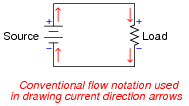Draw current arrows and voltage polarity marks ( and - symbols) next to each of the following components, the left group representing a battery, capacitor, and inductor all acting as energy sources and the right group representing a resistor, capacitor, and inductor all acting as energy loads: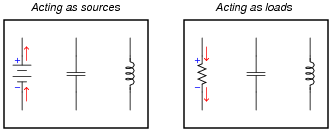• #### Question 6

Suppose this circuit were constructed, using a fully discharged capacitor (0 volts) and a voltmeter connected in parallel with it to measure its voltage: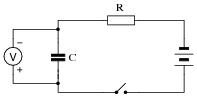What will happen to the capacitor’s voltage after the switch is closed? Be as precise as you can with your answer, and explain why it does what it does.

• #### Question 7

Suppose this circuit were constructed, using an inductor and an ammeter connected in series with it to measure its current:What will happen to the inductor’s current after the switch is closed? Be as precise as you can with your answer, and explain why it does what it does.

• #### Question 8

Graph both the capacitor voltage (EC) and the capacitor current (IC) over time as the switch is closed in this circuit. Assume the capacitor begins in a complete uncharged state (0 volts):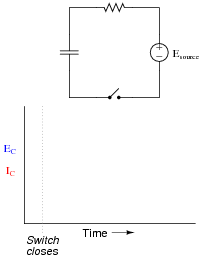• #### Question 9

Graph both the inductor voltage (EL) and the inductor current (IL) over time as the switch is closed in this circuit: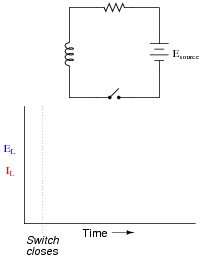• #### Question 10

There are many, many processes in the natural sciences where variables either grow (become larger) or decay (become smaller) over time. Often, the rate at which these processes grow or decay is directly proportional to the growing or decaying quantity. Radioactive decay is one example, where the rate of decay of a radioactive substance is proportional to the quantity of that substance remaining. The growth of small bacterial cultures is another example, where the growth rate is proportional to the number of live cells.

In processes where the rate of decay is proportional to the decaying quantity (such as in radioactive decay), a convenient way of expressing this decay rate is in terms of time: how long it takes for a certain percentage of decay to occur. With radioactive substances, the decay rate is commonly expressed as half-life: the time it takes for exactly half of the substance to decay: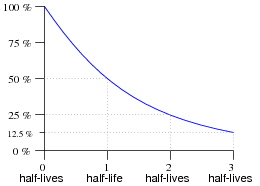In RC and LR circuits, decay time is expressed in a slightly different way. Instead of measuring decay rate in units of half-lives, we measure decay rate in units of time constants, symbolized by the Greek letter “tau” (τ).

What is the percentage of decay that takes place in an RC or LR circuit after one “time constant’s” worth of time, and how is this percentage value calculated? Note: it is not 50%, as it is for “half life,” but rather a different percentage figure.

Graph the curve of this decay, plotting points at 0, 1, 2, and 3 time constants:• #### Question 11

Suppose a capacitor is charged by a voltage source, and then switched to a resistor for discharging:Would a larger capacitance value result in a slower discharge, or a faster discharge? How about a larger resistance value? You may find the Öhm’s Law” equation for capacitance helpful in answering both these questions:

 i = C dv dt

Now consider an inductor, “charged” by a current source and then switched to a resistor for discharging:Would a larger inductance value result in a slower discharge, or a faster discharge? How about a larger resistance value? You may find the Öhm’s Law” equation for inductance helpful in answering both these questions:

 v = L di dt

• #### Question 12

Capacitors tend to oppose change in voltage, and so they may be considered “temporary voltage sources.” That is, they tend to hold a constant voltage over time, but they cannot do so indefinitely. Any motion of charge (current) will change the voltage of a capacitor.

Likewise, inductors may be considered “temporary current sources” because while they tend to hold current constant over time, they cannot do so indefinitely. Any application of voltage across a (perfect) inductor will alter the amount of current going through it.

Given the above characterizations, determine what resistance levels will result in the fastest discharge of energy for both the capacitive circuit and the inductive circuit. Considering each of the reactive components (C and L, respectively) as “temporary” power sources whose store of energy will drain over time, determine what value of R in each circuit will result in the quickest depletion of energy by making each source “work hardest:”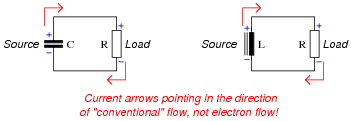Based on your answer to this question, explain how circuit resistance (R) affects the time constants (τ) of RC and of LR circuits.

• #### Question 13

While many students find it easy to understand how the value of R affects the time constant of a resistor-capacitor charging circuit (more R means slower charging; less R means faster charging), the opposite behavior of resistor-inductor circuits (less R means slower charging; more R means faster charging) seems incomprehensible:Resistor-capacitor circuit charging behavior probably makes more sense to students because they realize resistance controls charging current, which in turn directly effects how quickly the capacitor’s voltage may rise:

 i = C dv dt

Current (i) is proportional to the rate of change of voltage $$\frac{dv}{dt}$$. Since current is inversely proportional to resistance in a circuit powered by a voltage source, so must be the capacitor charging rate.

One way to help make the inductor charging circuit more sensible is to replace the series voltage-source/resistor combination with its Norton equivalent, a parallel current-source/resistor combination: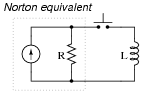Re-analyze the circuit in this form, and try to explain why more resistance makes the inductor charging time faster and less resistance makes the inductor charging time slower.

• #### Question 14

What value of resistor would need to be connected in series with a 33 μF capacitor in order to provide a time constant (τ) of 10 seconds? Express your answer in the form of a five-band precision resistor color code (with a tolerance of /- 0.1%).

• #### Question 15

What value of resistor would need to be connected in series with a 75 mH inductor in order to provide a time constant (τ) of 20 microseconds? Express your answer in the form of a five-band precision resistor color code (with a tolerance of /- 0.25%).

• #### Question 16

An electronic service technician prepares to work on a high-voltage power supply circuit containing one large capacitor. On the side of this capacitor are the following specifications:

 3000 WVDC 0.75 μF

Obviously this device poses a certain amount of danger, even with the AC line power secured (lock-out/tag-out). Discharging this capacitor by directly shorting its terminals with a screwdriver or some other piece of metal might be dangerous due to the quantity of the stored charge. What needs to be done is to discharge this capacitor at a modest rate.

The technician realizes that she can discharge the capacitor at any rate desired by connecting a resistor in parallel with it (holding the resistor with electrically-insulated pliers, of course, to avoid having to touch either terminal). What size resistor should she use, if she wants to discharge the capacitor to less than 1% charge in 15 seconds? State your answer using the standard 4-band resistor color code (tolerance = /- 10%).

• #### Question 17

The circuit shown here is called a relaxation oscillator. It works on the principles of capacitor charging over time (an RC circuit), and of the hysteresis of a gas-discharge bulb: the fact that the voltage required to initiate conduction through the bulb is significantly greater than the voltage below which the bulb ceases to conduct current.

In this circuit, the neon bulb ionizes at a voltage of 70 volts, and stops conducting when the voltage falls below 30 volts:Graph the capacitor’s voltage over time as this circuit is energized by the DC source. Note on your graph at what times the neon bulb is lit:

• #### Question 18

Replace the fixed-value resistor with a potentiometer to adjust the blinking rate of the neon lamp, in this relaxation oscillator circuit. Connect the potentiometer in such a way that clockwise rotation of the knob makes the lamp blink faster:• #### Question 19

Design a circuit with a three-position switch, where one position charges a capacitor, one position holds the capacitor’s voltage constant, and the last position discharges the capacitor. Provide independent, variable adjustments for charging and discharging time constants.

• #### Question 20

An intervalometer is a device that measures the interval of time between two events. Such devices are commonly used to measure the speed of projectiles, given a known distance between two sensors:A crude intervalometer may be constructed using two thin wires as sensors, which are broken by the passage of the projectile. The two wires are connected in an RC circuit as such:In order for this circuit to function properly as an intervalometer, which wire does the projectile need to break first? Explain why. Also, the voltmeter used in this instrument must be one with as high an input resistance as possible for best accuracy. Explain why this is necessary as well.

Which will produce a greater voltage indication after the test, a fast projectile or a slow projectile? Explain your answer.

• #### Question 21

Suppose we were measuring the interior temperature of an insulated box recently removed from a refrigerator, as it was being warmed by the ambient air around it: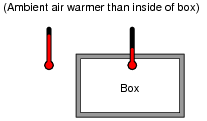Graphing the box’s temperature over time, we see a curve that looks something like this: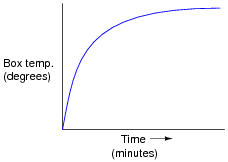An engineer approaches you and says she wants you to build an electrical circuit that models this thermal system. What kind of circuit would you consider building for the engineer, to make a realistic electrical analogue of the box’s temperature? Be as specific as you can in your answer.

• #### Question 22

Electromechanical relays are extremely useful devices, but they have their idiosyncrasies. One of them is a consequence of the fact that the relay’s coil acts as an inductor, storing energy in its magnetic field.

In the following circuit, a relay is used to switch power to a large electric motor, while being controlled by a light-duty pushbutton switch: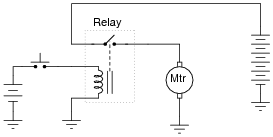The problem here is every time the pushbutton switch is released, the contacts arc significantly. This happens because the inductor releases all of its stored energy as a high-voltage spark across the opening contacts. Inductive kickback is the phrase commonly used to describe this effect, and over time it will prematurely destroy the switch.

An electronics technician understands the nature of the problem and proposes a solution. By connecting a light bulb in parallel with the relay coil, the coil’s energy now has a safer place to dissipate whenever the pushbutton switch contacts open: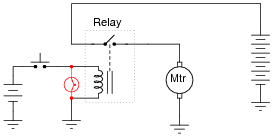Instead of that stored energy manifesting itself as a high-voltage arc at the switch, it powers the light bulb for a brief time after the switch opens, dissipating in a non-destructive manner.

However, the addition of the light bulb introduces a new, unexpected problem to the circuit. Now, when the pushbutton switch is released, the relay delays for a fraction of a second before disengaging. This causes the motor to övershoot” its position instead of stopping when it is supposed to.

Explain why this happens, with reference to the LR time constant of this circuit before and after the addition of the lamp.

• #### Question 23

An electrical testing laboratory has designed and built a high-current ßurge” power supply for testing the effects of large electrical currents on certain types of components. The basic idea is that a bank of capacitors are charged to a high voltage by a DC power source (through a current-limiting resistor to protect the power source from overcurrent damage), then quickly discharged through a switch to the test load:Due to the magnitude of the transient currents generated by the discharging capacitors, a mechanical switch (as suggested by the pushbutton symbol) is impractical. Instead, an air gap switch is constructed from two metal balls with a needle-point ïonizer” electrode between them. The ionizer electrode connects to a simple inductor/switch circuit that generates a high-voltage pulse sufficient to create a spark. The ionized air created in the spark provides a low-resistance path through the air which the 2000 volts from the capacitor bank can now traverse, thus completing the circuit for surge current between the capacitors and the test load:Suppose this surge power supply circuit failed to work after several months of good service. Nothing happens any more when the pushbutton switch is pressed and released. No spark is heard or seen in the air gap, and the test load receives no surge of current.

A voltmeter placed (carefully!) in parallel with the capacitor bank indicates a full charge voltage of 2035 volts, which is within normal parameters. Based on this information, identify these things:

Two components in the circuit that you know must be in good working condition.
Two components in the circuit that could possibly be bad, and the mode of their failure (either open or shorted).